# Scientific Method The Scientific Method The scientific method

• Slides: 14Scientific Method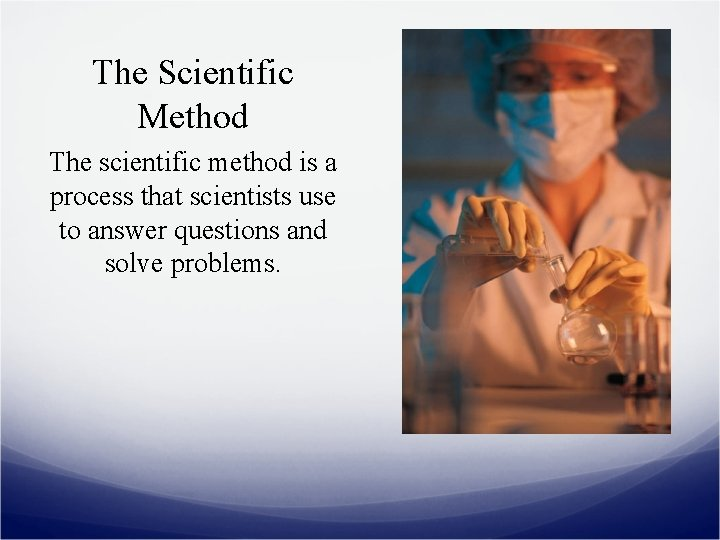The Scientific Method The scientific method is a process that scientists use to answer questions and solve problems.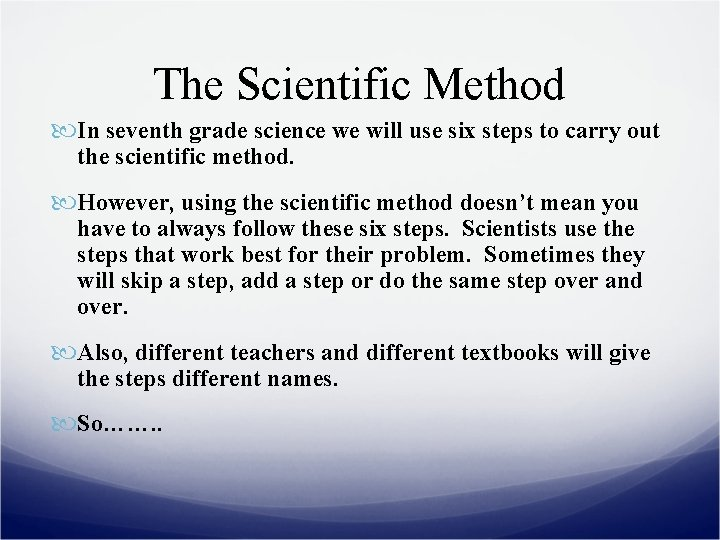The Scientific Method In seventh grade science we will use six steps to carry out the scientific method. However, using the scientific method doesn’t mean you have to always follow these six steps. Scientists use the steps that work best for their problem. Sometimes they will skip a step, add a step or do the same step over and over. Also, different teachers and different textbooks will give the steps different names. So……. .The Scientific Method • The important thing to remember about the scientific method is that whatever steps you use or what you call them, you are solving a problem using an organized, thoughtful, precise process.Steps of Scientific Method for Grade Science th 7 The following are the steps of the scientific method we will use in 7 th grade science: P = Problem I = Information H = Hypothesis E = Experiment R = Record Data or Results C = ConclusionProblem The problem is what you are trying to learn. It will always be in the form of a question. This question must be testable, which means you could set up an experiment to try to find an answer.Information In this step you gather information about the problem (question). Then you use that information to help write a hypothesis and decide how to set up your experiment.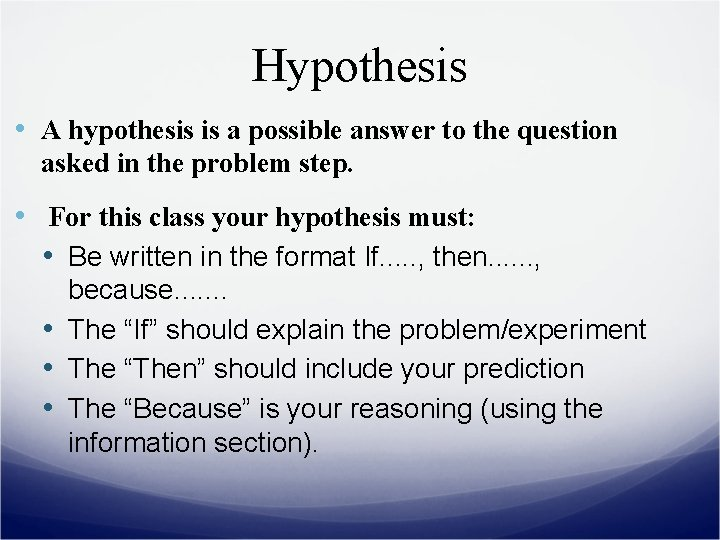Hypothesis • A hypothesis is a possible answer to the question asked in the problem step. • For this class your hypothesis must: • Be written in the format If. . . , then. . . , because. . . . • The “If” should explain the problem/experiment • The “Then” should include your prediction • The “Because” is your reasoning (using the information section).Hypothesis Example Problem: What ramp covering will cause a toy car to move down a ramp fastest? Carpet, Sandpaper or Glass? Hypothesis: If we send a toy car down three ramps covered in different materials, then the toy car will move down the ramp covered in glass fastest because glass will cause the least amount of friction between the ramp and the car tires.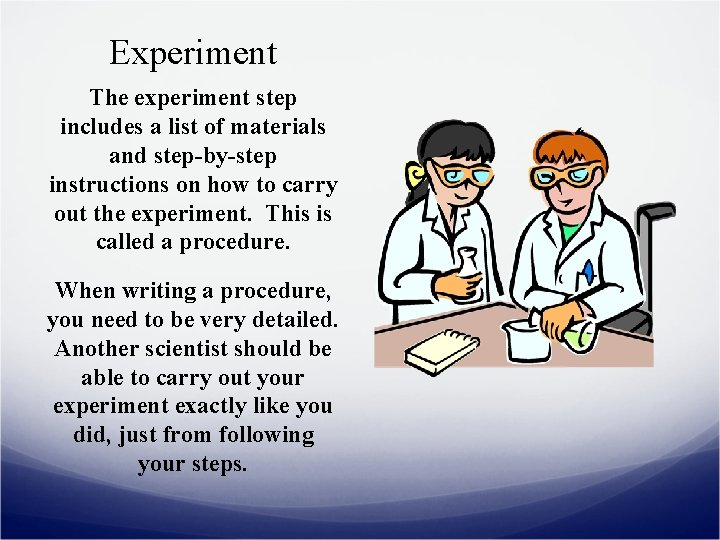Experiment The experiment step includes a list of materials and step-by-step instructions on how to carry out the experiment. This is called a procedure. When writing a procedure, you need to be very detailed. Another scientist should be able to carry out your experiment exactly like you did, just from following your steps.Results • In this step you carefully record the data collected in your experiment. • Observations are an important type of data. • Numerical data should be organized into tables. • Graphs are frequently made in this step to help make sense of data.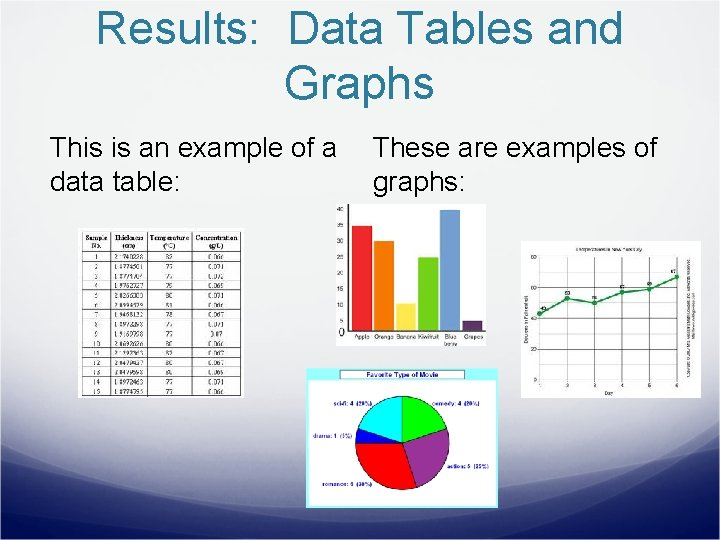Results: Data Tables and Graphs This is an example of a data table: These are examples of graphs: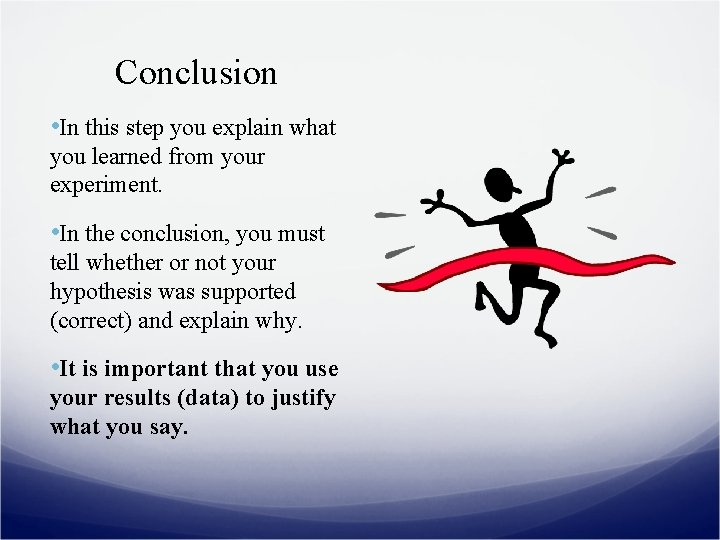Conclusion • In this step you explain what you learned from your experiment. • In the conclusion, you must tell whether or not your hypothesis was supported (correct) and explain why. • It is important that you use your results (data) to justify what you say.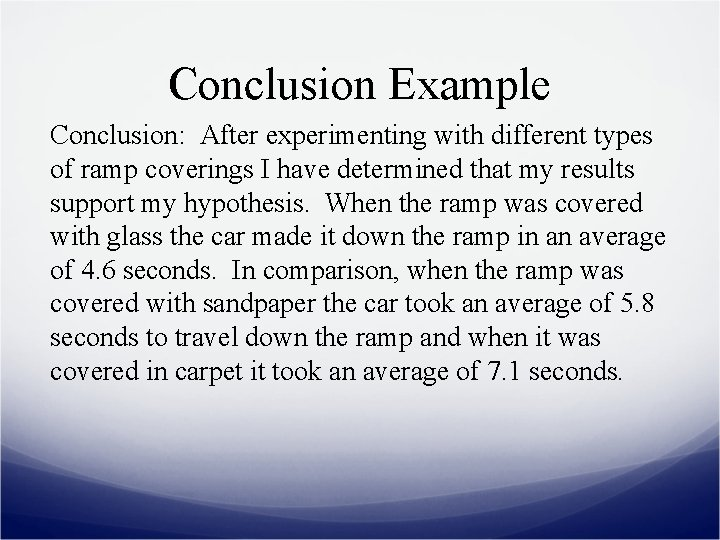Conclusion Example Conclusion: After experimenting with different types of ramp coverings I have determined that my results support my hypothesis. When the ramp was covered with glass the car made it down the ramp in an average of 4. 6 seconds. In comparison, when the ramp was covered with sandpaper the car took an average of 5. 8 seconds to travel down the ramp and when it was covered in carpet it took an average of 7. 1 seconds.